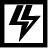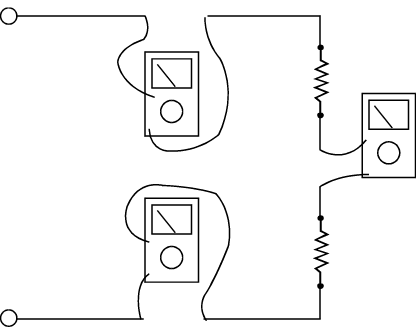# 1.4: Introduction to current measurements

•• Contributed by Camosun College

As you have seen, the placement of meter leads for voltage measurements is straightforward. The leads are simply connected across, or in parallel with, the points of voltage to be measured.

For current measurements, however, the process is slightly more complex. First, the circuit must be opened at the test points and the meter inserted in series at that opening (Figure $$\PageIndex{1}$$). The total current must flow through the meter. To allow the measurement to be made without disturbing the circuit itself, the current meter must have very little internal resistance.

This is where the beginner must be particularly alert. If the meter is inadvertently connected across a P.D. (potential difference) or in parallel with a component instead of in series, the small internal resistance will permit a very large current to flow through the meter. This will most certainly damage the meter severely and perhaps the circuit as well.With current measurements, shutting off the power before connecting the meter is essential. You will be disconnecting one end of a wire or component in order to connect the meter in series. If you leave the power on, you could easily receive a dangerous shock or do damage to the circuit.

On meters with manual range selection, start with the highest current setting and work your way down.Figure $$\PageIndex{1}$$: Using a DMM to measure current (CC BY-NC-SA; BC Industry Training Authority)

## Current Measurement Procedures

Follow the steps below to measure current:

1. Turn off the power to the circuit that will be measured.
2. Open the circuit by disconnecting or unsoldering a connection at a point where you wish to measure current.
3. Select the DC or AC amps function by turning the function switch to DC or AC amps.
4. Plug the test probes into the appropriate probe jacks. Note that the jacks used may not be the same ones used to measure volts.
5. Connect the tips of the probes across the break in the circuit as shown in Figure $$\PageIndex{2}$$ so that the current to be measured flows through the meter. Note that this is a series connection. Never connect the ammeter in parallel with the source or load, as this will cause a short circuit and damage the meterFigure $$\PageIndex{2}$$: Ammeter connections to measure the same current at different points in a circuit (CC BY-NC-SA; BC Industry Training Authority)
1. Turn the circuit power back on.
2. View the reading on the display unit. Be sure to note the unit of measurement.
3. Switch power off and disconnect meter leads from the circuit.
4. If testing is finished at this test point, restore the circuit by reclosing the connection. When current measurements are complete, turn the function switch to the OFF position and remove the test leads.

Some types of DMMs have a clamp-on ammeter or tong tester. These ammeters have two springloaded expandable jaws that allow you to clamp around a single conductor (Figure $$\PageIndex{3}$$). This feature allows you to measure the magnetic field created by the current flowing through the wire to give an ampere reading without having to make physical contact or disconnect the circuit.Figure $$\PageIndex{3}$$: Using clamp-on ammeter (CC BY-NC-SA; BC Industry Training Authority).